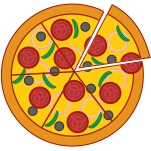# Numerator - Definition with Examples

The Complete K-5 Math Learning Program Built for Your Child

• 40 Million Kids

Loved by kids and parent worldwide

• 50,000 Schools

Trusted by teachers across schools

• Comprehensive Curriculum

Aligned to Common Core

What is Numerator?

When numbers are written in the form of a fraction, it can be represented as  a, where a is the numerator and b is the denominator. For example, 45 is a fraction, and the line separating the numbers 4 and 5 is the fraction bar. Here the number above the fraction bar is the numerator, and the one below the fraction bar is the denominator. A numerator represents the number of parts out of the whole, which is the denominator.

Here is an example of a numerator:Out of a pizza having 6 slices, Rena gets 1 slice. That means the fraction for Rena is 1, where 1 is the numerator. In other words, she gets one-sixth of the pizza.

Likewise, in 4, 4 is the numerator; in the fraction 2549 , 25 is the numerator and so on. So anything that is above the fraction bar or on the top in a fraction is the numerator.